## Area and Perimeter - Problem Sums

Practice Unlimited Questions

#### 1. Adriel ran 5 rounds of a square ground of side 70 m. Find the total distance he ran.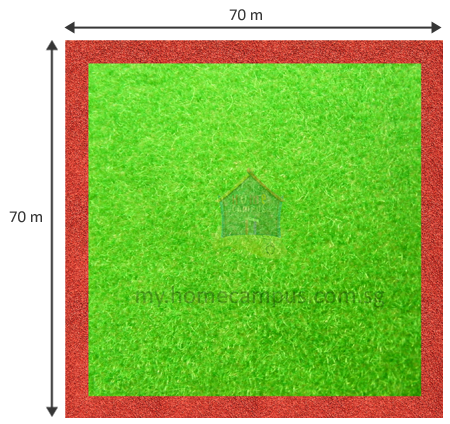Step 1:
Distance covered in 1 round of the square ground
=  Perimeter of the square ground
=  70  +  70  +  70  +  70
=  280 m

Step 2:
Distance covered in 5 rounds of the square ground
=  5  ×  280
=  1400 m

He ran a total distance of 1400 m.

#### 2. Mrs. Rao gives one rectangular card of sides 18 cm by 14 cm to each of her 7 pupils. She wants her pupils to glue a ribbon around the border of their own card. Find the length of the ribbon they will need altogether.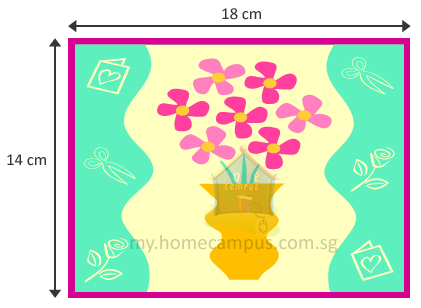Step 1:
Perimeter of 1 card
=  18 + 14 + 18 + 14
=  64 cm

Step 2:
Each pupil needs 64 cm of ribbon for their card.
Hence, 7 pupils will need
=  7 × 64
=  448 cm

They will need 448 cm of ribbon altogether.

#### 3. Lila has 9 square stamps of side 3 cm each. She glues them onto an envelope to form a bigger square. What area of the envelope does the bigger square cover?

The 9 stamps can be arranged to form a square as shown below.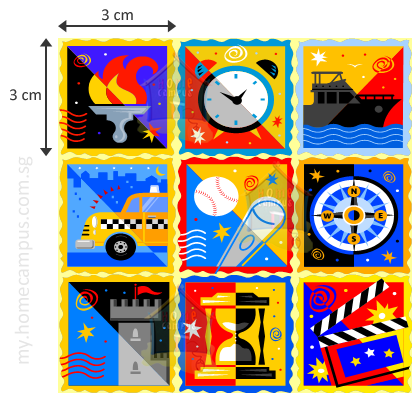Step 1:
Side of the square formed
=  3  +  3  +  3
=  9 cm

Step 2:
Area of the square formed
=  9  ×  9
=  81 cm2

The area of the envelope that the bigger square covers is 81 cm2.

#### 4. Mrs. Bell cuts a 25 cm by 6 cm cloth into 5 equal pieces. What is the area of each piece?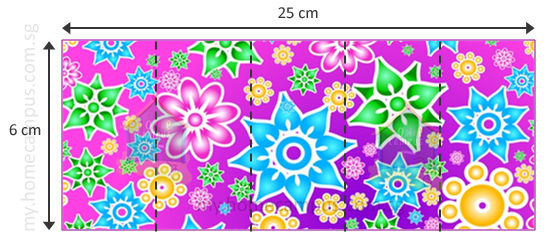Step 1:
Area of the cloth
=  25  ×  6
=  150 cm2

Step 2:
Area of each cloth
=  150  ÷  5
=  30 cm2

The area of each piece is 30 cm2.

#### 5. You have 4 squares each of side 8 cm. Join the squares to form a bigger four-sided figure. What is the longest possible perimeter that you can get?

Using the 4 squares we can form either a bigger square (Figure A) or a rectangle (Figure B).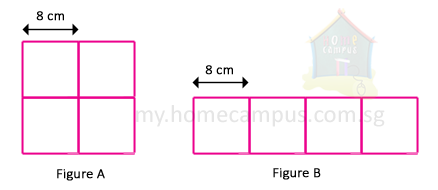Figure A:
Side of the big square
=  Side of the small square  +  Side of the small square
=  8  +  8
=  16 cm

Perimeter of the big square
=  16  +  16  +  16  +  16
64 cm

Figure B:
Length of the rectangle
=  8  +  8  +  8  +  8
=  32 cm